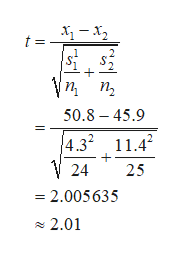# Use the given statistics to complete parts​ (a) and​ (b). Assume that the populations are normally distributed.​(a) Test whethermu 1μ1greater than>mu 2μ2at thealphaα=0.01level of significance for the given sample data.​(b) Construct a99​%confidence interval aboutmu 1μ1minus−mu 2μ2.  Population 1Population 2 n2425x overbarx50.845.9s4.311.4​(a) Identify the null and alternative hypotheses for this test.  A.H0​:mu 1μ1greater than>mu 2μ2H1​:mu 1μ1equals=mu 2μ2 B.H0​:mu 1μ1equals=mu 2μ2H1​:mu 1μ1less than<mu 2μ2 C.H0​:mu 1μ1less than<mu 2μ2H1​:mu 1μ1equals=mu 2μ2 D.H0​:mu 1μ1not≠mu 2μ2Upper H 1H1​:mu 1μ1equals=mu 2μ2 E.H0​:mu 1μ1=mu 2μ2H1​:mu 1μ1greater than>mu 2μ2 F.H0​:mu 1μ1equals=mu 2μ2H1​:mu 1μ1not equals≠mu 2μ2Find the test statistic for this hypothesis test. 2.01​(Round to two decimal places as​ needed.)Determine the​ P-value for this hypothesis test. 0.027​(Round to three decimal places as​ needed.)State the conclusion for this hypothesis test.  A.RejectH0.Thereis not sufficient evidence at thealphaα=0.01level of significance to conclude thatmu 1μ1greater than>mu 2μ2. B.RejectH0.Thereis sufficient evidence at thealphaα=0.01level of significance to conclude thatmu 1μ1greater than>mu 2μ2. C.Do not rejectH0.Thereis not sufficient evidence at thealphaα=0.01level of significance to conclude thatmu 1μ1greater than>mu 2μ2. D.Do not rejectH0.Thereis sufficient evidence at thealpha=0.01level of significance to conclude thatmu 1μ1greater than>mu 2μ2.​(b) The99%confidence interval aboutmu 1μ1minus−mu 2μ2is the range from a lower bound of_to an upper bound of_.​(Round to three decimal places as​ needed.)

Question
1 views

 Population 1 Population 2 Use the given statistics to complete parts​ (a) and​ (b). Assume that the populations are normally distributed. ​(a) Test whether mu 1μ1greater than>mu 2μ2 at the alphaα=0.01 level of significance for the given sample data. ​(b) Construct a 99​% confidence interval about mu 1μ1minus−mu 2μ2. 24 25 50.8 45.9 4.3 11.4
​(a) Identify the null and alternative hypotheses for this test.

A.
H0​:
mu 1μ1greater than>mu 2μ2
H1​:
mu 1μ1equals=mu 2μ2

B.
H0​:
mu 1μ1equals=mu 2μ2
H1​:
mu 1μ1less than<mu 2μ2

C.
H0​:
mu 1μ1less than<mu 2μ2
H1​:
mu 1μ1equals=mu 2μ2

D.
H0​:
mu 1μ1not≠mu 2μ2
Upper H 1H1​:
mu 1μ1equals=mu 2μ2

E.
H0​:
mu 1μ1=mu 2μ2
H1​:
mu 1μ1greater than>mu 2μ2

F.
H0​:
mu 1μ1equals=mu 2μ2
H1​:
mu 1μ1not equals≠mu 2μ2
Find the test statistic for this hypothesis test.

2.01
​(Round to two decimal places as​ needed.)
Determine the​ P-value for this hypothesis test.

0.027
​(Round to three decimal places as​ needed.)
State the conclusion for this hypothesis test.

A.
Reject
H0.
There
is not sufficient evidence at the
alphaα=0.01
level of significance to conclude that
mu 1μ1greater than>mu 2μ2.

B.
Reject
H0.
There
is sufficient evidence at the
alphaα=0.01
level of significance to conclude that
mu 1μ1greater than>mu 2μ2.

C.
Do not reject
H0.
There
is not sufficient evidence at the
alphaα=0.01
level of significance to conclude that
mu 1μ1greater than>mu 2μ2.

D.
Do not reject
H0.
There
is sufficient evidence at the
alpha=0.01
level of significance to conclude that
mu 1μ1greater than>mu 2μ2.
​(b) The
99%
mu 1μ1minus−mu 2μ2
is the range from a lower bound of_
to an upper bound of_.
(Round to three decimal places as​ needed.)
check_circle

Step 1

Let four-year colleges be the first sample and the two-year colleges be the second sample.

Option E is correct.

Hypotheses for the test is given below:

Step 2

This test is a right-tailed test.

Test statistic for t-test:

Since population standard deviations are unknown, the appropriate test is two sample t-test.

Here, the sample means of 1st and 2nd samples are 50.8 and 45.9 respectively.

Sample standard deviations of 1st and 2nd samples are 4.3 and 11.4 respectively.

Sample size of 1st and 2nd samples are 24 and 25 respectively.

The pooled standard deviation can be calculated as follows:

The test statistic for t-test is calculated as given below.help_outlineImage Transcriptioncloseх — х, t = Уп, п, 50.8 45.9 4.32 11.42 24 25 = 2.005635 2.01 fullscreen
Step 3

The value of test statistic is 2.5.

Computation of P-value:

The right tailed P-value for the t-test at 47 degrees of freedom is approximately 0.025 whi...

### Want to see the full answer?

See Solution

#### Want to see this answer and more?

Solutions are written by subject experts who are available 24/7. Questions are typically answered within 1 hour.*

See Solution
*Response times may vary by subject and question.
Tagged in

### Hypothesis Testing